# AMP II.2 – General Feedback Theory

### Introduction

Before diving practical amplifier circuits, we need to address the underlying theory behind feedback amplifiers.  This chapter will contain the essential formulas and vocabulary to be able to move on to more practical topics.

### A Little Bit of Control Theory

If you’ve studied automation, electronics, or mechanical engineering, chances are you’ve come across control theory.  It is an extremely versatile tool that can be applied to countless scientific domains, including electronics.  We can represent our amplifier circuit by a black box with a gain of A.  An open-loop amplifier would then be drawn as:The transfer function of this amplifier is simplywould represent the active device in our amplifier, typically a transistor or an op-amp.

A closed-loop amplifier, featuring feedback, would have a portion of its output fed back through its input, like so:The above diagram is a representation of a feedback amplifier.  While you will sometimes see the output directly coupled to the input with no circuitry in between, most often thee will be components between the output and the input.  These components create the feedback circuitry of the amplifier.  The transfer function of this feedback circuitry is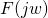.

What changes in a feedback amplifier?  Well, the input signal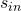isn’t fed directly to the amplifier, but is instead summed with the signal coming from the feedback network.  This signal is.

Thus, what comes out of the summation node is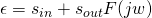.  We also know that.

Substitutingin the expression of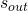gives: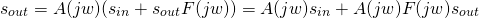We then have the following expression:Thus, the transfer function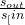becomes:The quantityis known as the loop gain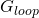, and is a fundamental characteristic of feedback cirtcuits.

### Ideal Feedback

Using the above transfer function, we can derive: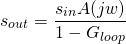Since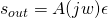, we have: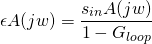Let’s recap the two main equations we’ve found:

(1)(2)Notice what happens when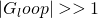:

•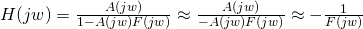•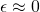Notice how for a high loop gain, the transfer function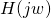no longer depends on the amplifier’s characteristics, but only on its feedback network.  Since the amplifier’s characteristicrefers to the active device’s characteristic, having a transfer function independent ofmeans it is no longer subject to semiconductor variability.  Furthermore, the feedback network described byusually consists of passive components such as resistors.  Having an amplifier whose transfer function only depends on passive components like resistors makes it much easier to control gain and reproduce results.  This is one major advantage of closed loop design.

When loop gain is infinite, we consider the feedback as ideal.  Our loop gain will of course never be infinite, but if we want our equations and designs to work, we need to ensure that the loop gainis high enough to ensure that our transfer function indeed only depends on the feedback network.  Generally, a loop gain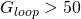is ideal.  Less than 10 and the real results will stray too far from our ideal version.

### Positive and Negative Feedback

Let’s take a look at the transfer functionagain: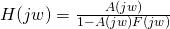, or, can be either positive or negative.  Ifis positive, thenandhave the same sign, andwill be greater than the input signal (in absolute value).  In this case, feedback tends to increase the output.  As the cycle repeats, the output will grow larger and larger until the circuit saturates.  This is called positive feedback.  The saturation usually occurs pretty, and very soon the output will simply be the maximum value the supply voltage will allow.  There are some cases when positive feedback is useful.  Oscillators, which we will cover in another section, come to mind.  But for amplifiers, positive feedback is useless.

Ifis negative, thenandhave different signs, andwill be smaller than the input signal.  Here feedback is used to decrease the signal fed to the amplifier.  This is negative feedback.

### The Magic Quantity

Let’s differentiate our transfer functionwith regards to: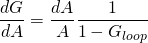What does this mean?  It means that if our active device’s propertiesvary by a certain amount, our final transfer function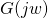will only vary by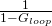that amount, a huge reduction ifis high enough.  Semiconductor variability is effectively cancelled by using negative feedback.  Of course, there is a price to pay.  Looking at the transfer function, we can see that the gain is also reduced by a factor of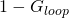.

When studying feedback circuits, the quantitywill appear very often.  It is the magic quantity of feedback amplifiers.

### Conclusion

At the end of this chapter, you should understand:

• the transfer function of a feedback amplifier• the loop gain of a feedback circuit is• for a high enough loop gain (ideal negative feedback):, thereby eliminating active device varibility
• for a high enough loop gain (ideal negative feedback):• the quantity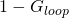will often appear when studying feedback circuits